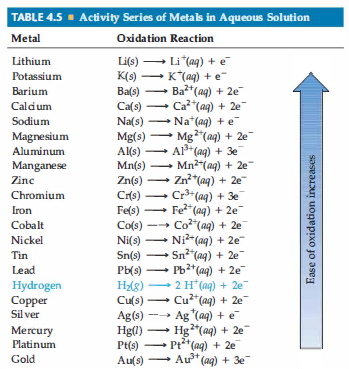# Problem: Based on the activity series (Table 4.5), what is the outcome (if any) of each of the following reactions?(e) H2(g) + CuCl2(aq) →

🤓 Based on our data, we think this question is relevant for Professor Chiucchi's class at OSU.

###### Problem Details

Based on the activity series (Table 4.5), what is the outcome (if any) of each of the following reactions?

(e) H2(g) + CuCl2(aq) →